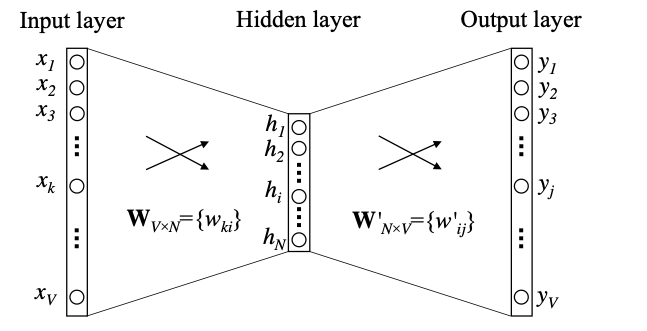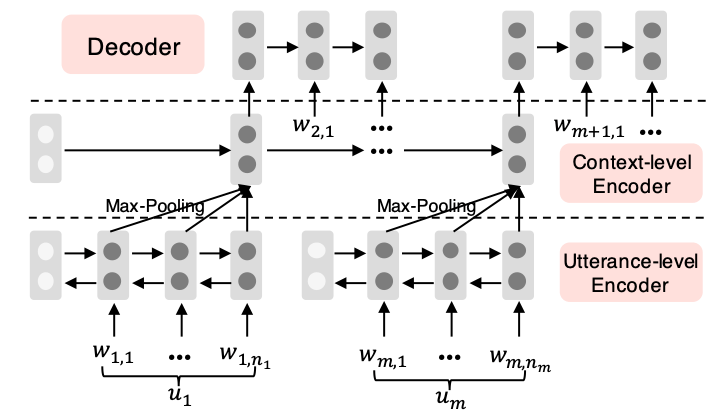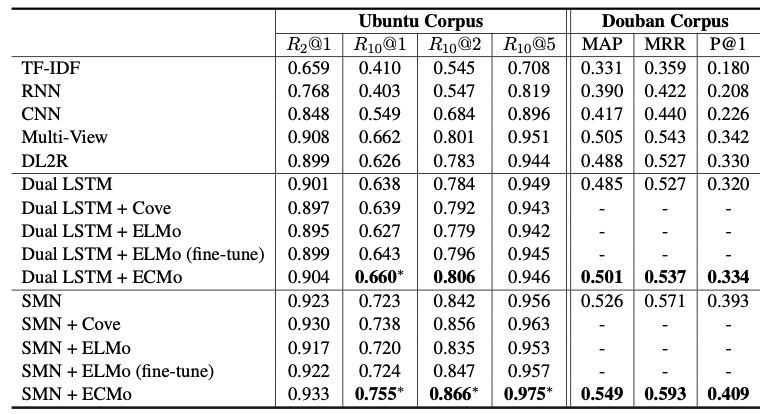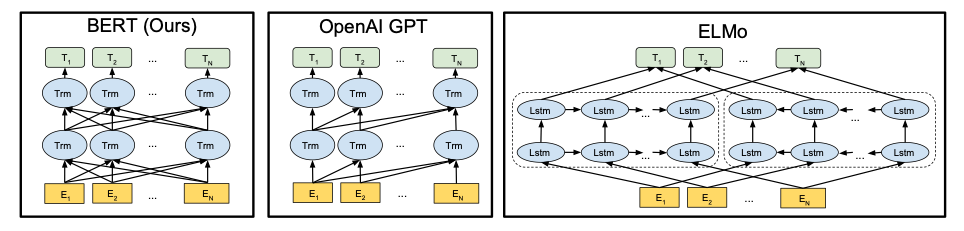# 从ECMo、Bert看Word Embedding

ECMo = embeddings from a conversation model

## word2Vec

word2Vec 思路其实和我们之前用到的大部分模型一致• 一个输入 多个输出 Skip-gram
• 多个输入 一个输出 Cbow

P ( w n = w ∣ w 1 , ⋯ &ThinSpace; , w n − 1 ) = σ ( v w ⋅ ∑ i = 1 n − 1 C i v w i + b w ) P(w_n=w|w_1,\cdots,w_{n-1})=\sigma(v_w \cdot \sum\limits_{i=1}^{n-1} C_i v_{w_i} + b_w) 简化为 P ( w n = w ∣ w 1 , ⋯ &ThinSpace; , w n − 1 ) = σ ( 1 n − 1 v w ⋅ ∑ i = 1 n − 1 v ~ w i ) P(w_n=w|w_1,\cdots,w_{n-1})=\sigma(\frac{1}{n-1} v_w \cdot \sum\limits_{i=1}^{n-1} \tilde{v}_{w_i})

负采样是解决SoftMax维数太大 计算效率低的问题 在计算SoftMax的时候除了一个正例之外 随机采样几个负样本 只要模型能中这几个样本中训练出正例就行了

log ⁡ σ ( v w 0 T v w I ) + ∑ i = 1 K E w i [ log ⁡ σ ( − v w i T v w I ) ] \log\sigma(v^{T}_{w_0}v_{w_I})+\sum\limits_{i=1}^KE_{w_i}[\log\sigma(-v^{T}_{w_i}v_{w_I})]

## ECMop ( u n + 1 ∣ u 1 , . . . , u n ) = p ( w n + 1 , 1 ∣ h n s ) ∏ t = 2 T n + 1 p ( w n + 1 , t ∣ h n s , w n + 1 , 1 , . . . , w n + 1 , t − 1 ) p(u_{n+1}|u_1,...,u_n)=p(w_{n+1,1}|h^s_n)\prod\limits_{t=2}^{T_{n+1}}p(w_{n+1,t}|h^s_n,w_{n+1,1},...,w_{n+1,t-1})

h t d = G R U d ( h t − 1 d , e n + 1 , t − 1 ) h^d_t=GRU_d(h^d_{t-1},e_{n+1,t-1}) , I I w n + 1 , t II_{w_{n+1,t}} 为one-hot## Bert

bert已然是当今学界的热点

Bert相当于在预处理阶段 把dataset中 字符级别、词级别、句子级别 甚至句子间所有特征抓取到### 深度双向Encoding

Bert为了达到这种深层双向Encoding的功能 做了一件我们从小都在做的事情 完形填空

• 80% 概率 用[mask]替换单词
• 10% 概率 用随机采样的单词替换
• 10% 概率不替换

### 句粒度

Embedding从最开始的One-hot 到从NN中间参数获取的word2Vec 再到双向Encoding的ELMo 最后到全能的Bert

## Reference

12-09207202-20877
03-04
07-288398
03-183895
04-15121